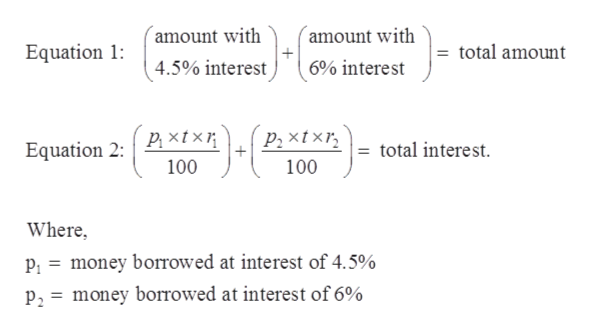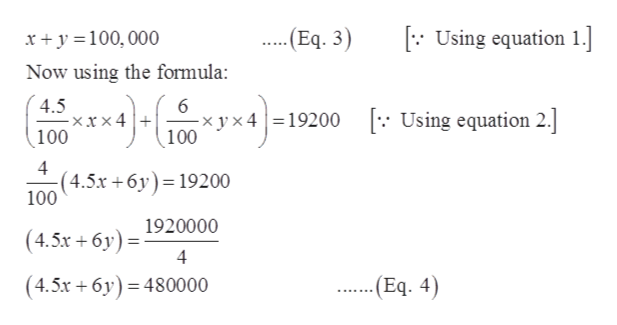# Juan borrows \$100,000 to pay for medical school. He borrows part of the money from the school whereby he will pay 4.5% simple interest. He borrows the rest of the money through a government loan that will charge him 6% interest. In both cases, he is not required to pay off the principal or interest during his 4 yr of medical school. However, at the end of 4 yr, he will owe a total of \$19,200 for the interest from both loans. How much did he borrow from each source?

Question
4 views

Juan borrows \$100,000 to pay for medical school. He borrows part of the money from the school whereby he will pay 4.5% simple interest. He borrows the rest of the money through a government loan that will charge him 6% interest. In both cases, he is not required to pay off the principal or interest during his 4 yr of medical school. However, at the end of 4 yr, he will owe a total of \$19,200 for the interest from both loans. How much did he borrow from each source?

check_circle

Step 1

Given:

Juan borrows \$100,000 to pay for medical school. He borrows part of the money from the school whereby he will pay 4.5% simple interest. He borrows the rest of the money through a government loan that will charge him 6% interest. In both cases, he is not required to pay off the principal or interest during his 4 yr of medical school. However, at the end of 4 yr, he will owe a total of \$19,200 for the interest from both loans. How much did he borrow from each source?

Step 2

Let there are two unknown quantity in the question. So, let assume first amount is x and another be y.help_outlineImage Transcriptionclose(amount with (amount with Equation 1: total amount 4.5% interest 6% interest р, xtxи P2 xt xr, = total interest. Equation 2: 100 100 Where, P; = money borrowed at interest of 4.5% p2 = money borrowed at interest of 6% fullscreen
Step 3

Now forming the two ...help_outlineImage Transcriptionclose[: Using equation 1. -(Eq. 3) x+ y =100,000 Now using the formula: 4.5 - x 100 x y x 4 =19200 100 : Using equation 2. x × 4 4 (4.5x +6y)= 19200 100 1920000 (4.5x + 6y) =: 4 ...(Eq. 4) (4.5x + 6y) = 480000 fullscreen

### Want to see the full answer?

See Solution

#### Want to see this answer and more?

Solutions are written by subject experts who are available 24/7. Questions are typically answered within 1 hour.*

See Solution
*Response times may vary by subject and question.
Tagged in

### Equations and In-equations